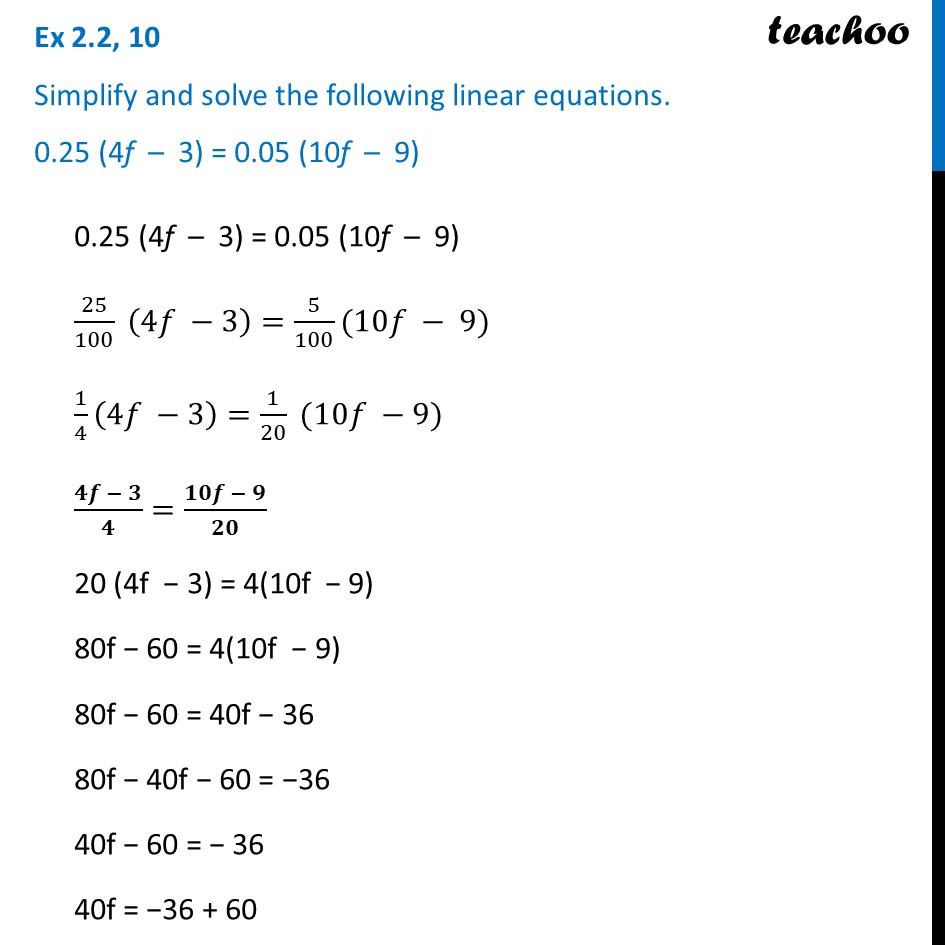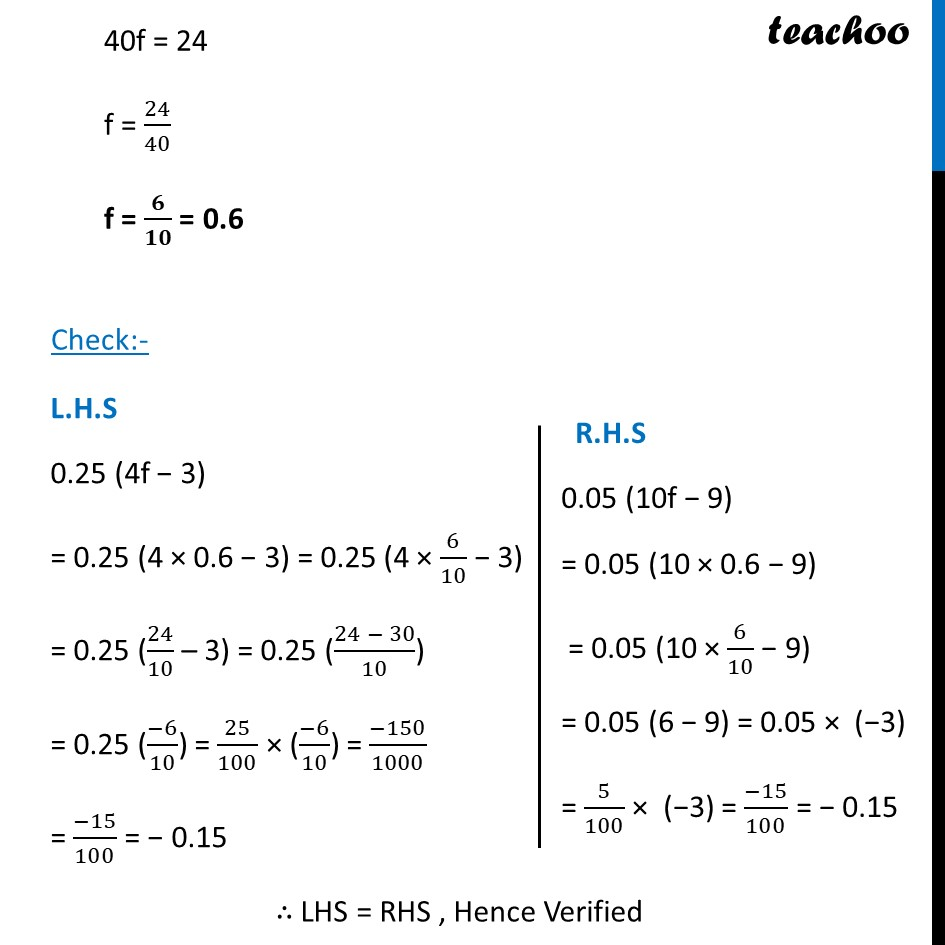Ex 2.2

Chapter 2 Class 8 Linear Equations in One Variable
Serial order wiseLearn in your speed, with individual attention - Teachoo Maths 1-on-1 Class

### Transcript

Ex 2.2, 10 Simplify and solve the following linear equations. 0.25 (4f – 3) = 0.05 (10f – 9)0.25 (4f – 3) = 0.05 (10f – 9) 25/100 (4𝑓 −3)=5/100(10𝑓 − 9) 1/4 (4𝑓 −3)=1/20 (10𝑓 −9) (𝟒𝒇 − 𝟑)/𝟒=(𝟏𝟎𝒇 − 𝟗)/𝟐𝟎 20 (4f − 3) = 4(10f − 9) 80f − 60 = 4(10f − 9) 80f − 60 = 40f − 36 80f − 40f − 60 = −36 40f − 60 = − 36 40f = −36 + 60 40f = 24 f = 24/40 f = 𝟔/𝟏𝟎 = 0.6 Check:- L.H.S 0.25 (4f − 3) = 0.25 (4 × 0.6 − 3) = 0.25 (4 × 6/10 − 3) = 0.25 (24/10 – 3) = 0.25 ((24 − 30)/10) = 0.25 ((−6)/10) = 25/100 × ((−6)/10) = (−150)/1000 = (−15)/100 = − 0.15 R.H.S 0.05 (10f − 9) = 0.05 (10 × 0.6 − 9) = 0.05 (10 × 6/10 − 9) = 0.05 (6 − 9) = 0.05 × (−3) = 5/100 × (−3) = (−15)/100 = − 0.15 ∴ LHS = RHS , Hence Verified